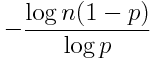Over a million developers have joined DZone.{{announcement.body}}
{{announcement.title}}

DZone's Guide to

# The Probability of Long Runs

· Big Data Zone ·
Free Resource

Comment (0)

Save
{{ articles.views | formatCount}} Views

Cloudera Data Flow, the answer to all your real-time streaming data problems. Manage your data from edge to enterprise with a no-code approach to developing sophisticated streaming applications easily. Learn more today.

Suppose you’ve written a program that randomly assigns test subjects to one of two treatments, A or B, with equal probability. The researcher using your program calls you to tell you that your software is broken because it has assigned treatment A to seven subjects in a row.

You might argue that the probability of seven A’s in a row is 1/2^7 or about 0.008. Not impossible, but pretty small. Maybe the software is broken.

But this line of reasoning grossly underestimates the probability of a run of 7 identical assignments. If someone asked the probability that the next 7 assignments would all be A’s, then 1/2^7 would be the right answer. But that’s not the same as asking whether an experiment is likely to see a run of length 7 because run could start any time, not just on the next assignment. Also, the phone didn’t ring out of the blue: it rang precisely because there had just been a run.

Suppose you have a coin that has probability of heads p and you flip this coin n times. A rule of thumb says that the expected length of the longest run of heads is aboutprovided that n(1-p) is much larger than 1.

So in a trial of n = 200 subjects with p = 0.5, you’d expect the longest run of heads to be about seven in a row. When p is larger than 0.5, the longest expected run will be longer. For example, if p = 0.6, you’d expect a run of about 9.

The standard deviation of the longest run length is roughly 1/log(1/p), independent of n. For coin flips with equal probability of heads or tails, this says an approximate 95% confidence interval would be about 3 either side of the point estimate. So for 200 tosses of a fair coin, you’d expect the longest run of heads to be about 7 ± 3, or between 4 and 10.

The following Python code gives an estimate of the probability that the longest run is between a and b inclusive, based on an extreme value distribution.

def prob(a, b, n, p):
r = -log(n*(1-p))/log(p)
cdf = lambda x: exp(- p**x )
return cdf(b + 1 - r) - cdf(a - r)

What if you were interested in the longest run of head or tails? With a fair coin, this just adds 1 to the estimates above. To see this, consider a success to be when consecutive coins turn up the same way. This new sequence has the same expected run lengths, but a run of length m in this sequence corresponds to a run of length m + 1 in the original sequence.

For more details, see “The Surprising Predictability of Long Runs” by Mark F. Schilling, Mathematics Magazine 85 (2012), number 2, pages 141–149.

Cloudera Enterprise Data Hub. One platform, many applications. Start today.

Topics:

Comment (0)

Save
{{ articles.views | formatCount}} Views

Published at DZone with permission of

Opinions expressed by DZone contributors are their own.

# {{ parent.title || parent.header.title}}

{{ parent.tldr }}

{{ parent.urlSource.name }}